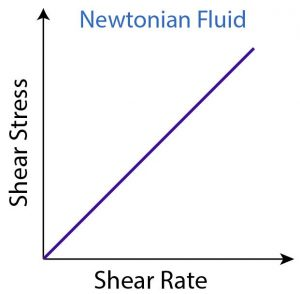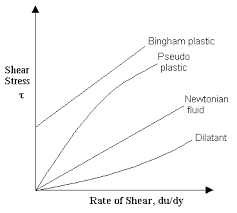# Viscosity and rheology: Newtonian system, Law of flow, Kinematic viscosity, Non Newtonian systems and MCQs for GPAT, NIPER, Pharmacist and Drug Inspector exam

## Viscosity and rheology: Newtonian system, Law of flow, Kinematic viscosity, Non Newtonian systems and MCQs for GPAT, NIPER, Pharmacist and Drug Inspector exam

The term ‘rheology’ was derived from the Greek words rheo (flow) and logos (science) and is used to describe the flow of liquids and the deformation of solids. Viscosity is an expression of the resistance of a fluid to flow: the higher the viscosity, the greater is the resistance.

Importance of Rheology in Pharmacy:

Manufacturers of medicinal and cosmetic creams, pastes, and lotions must be capable of producing products with acceptable consistency and smoothness and reproducing these qualities each time a new batch is prepared.

Rheology is involved in:

• the mixing and flow of materials, their packaging into containers, and
• their removal prior to use, whether this is achieved by pouring from a bottle, extrusion
• from a tube, or passage through a syringe needle.

Newtonian systems: Newtonian fluid is a fluid in which the viscous stresses arising from its flow, at every point, are linearly proportional to the local strain rate—the rate of change of its deformation over time. Water, air, alcohol, glycerol, and thin motor oil are all examples of Newtonian fluids over the range of shear stresses and shear rates encountered in everyday life.

Newton’s Law of Flow: Newton was the first to study now properties of liquids in a quantitative way. Newton’s Law of Flow states that the shear stress between adjacent fluid layers is proportional to the velocity gradient between two layers or shear rate.

Newtonian law of viscosityShearing stress is directly proportional to shearing rate.

• The difference in the velocity gradient (dv) between two plan of liquid separated by distance (dx) is the rate of shear (dv/dx) and it symbol is G.
• The force per unit area required to bring about flow is called the shearing stress ant its given a symbol F.
•Fig 1 – Newtonian rheogram (taken from types of flow and rheology models of drilling mud)

Two-plate model:

The two-plates model provides a mathematical description for viscosity. There are two plates with fluid placed in-between. The lower plate does not move. The upper plate drifts aside very slowly and subjects the fluid to a stress, which is parallel to its surface: the shear stress. The force applied to the upper plate divided by this plate’s area defines the shear stress (F’/A). Force/area results in the unit N/m², which is named Pascal [Pa].

The two-plate model allows for calculating another parameter: the shear rate (dv/dr). The shear rate is the velocity of the upper plate divided by the distance between the two plates. Its unit is [1/s] or reciprocal second [s-1].

Dynamic viscosity is shear stress divided by shear rate.

F/A = ndv/dr

where η is the coefficient of viscosity or simply viscosity or absolute viscosity or dynamic viscosity.

Ƞ = F/G

For a Newtonian system, a representative flow curve or rheogram, by plotting F versus G is shown below where a straight line passing through the origin is obtained.

The CGS unit of viscosity is the poise (dyne sec/cm2 or g cm- 1 sec- 1), defined as the shearing force required producing a velocity of 1cm/sec between two parallel planes of liquid each 1 cm2 in area and separated by a distance of 1 cm.

Fluidity – Ø is defined as the reciprocal of viscosity Ø = 1/ Ƞ

Kinematic viscosity: It is the absolute viscosity divided by the density of the liquid, Ƿ at a specific temperature.

Kinematic viscosity = Ƞ/ Ƿ

The cgs unit of kinematic viscosity is stoke (s) or cm2/sec and centistokes(cs) and SI unit of kinematic viscosity is m2/sec.

Effect of temperature: Apart from the shear rate, temperature strongly influences a fluid’s viscosity. A substance’s viscosity decreases with increasing temperature. As temperature is raised, the (the reciprocal of viscosity) increases with temperature. This inversely proportional relation applies Any change in temperature always influences viscosity, but for different fluids, of this influence varies. Even a 1 K (1 °C) temperature increase can raise the viscosity.

Temperature Dependence and the Theory of Viscosity- Whereas the viscosity of a gas increases with temperature, that of a liquid decreases as temperature is raised, and the fluidity of a liquid (the reciprocal of viscosity) increases with temperature. The dependence of the viscosity of a liquid on temperature is expressed approximately for many substances by an equation analogous to the Arrhenius equation of chemical kinetics:

Ƞ = AeE.RT

where A is a constant depending on the molecular weight and molar volume of the liquid and Ev is an activation energy required to initiate flow between molecules.

Non-newtonian system: non-Newtonian fluid is a fluid that does not follow Newton’s law of viscosity.

In a non-Newtonian fluid, the relation between the shear stress and the strain rate is nonlinear, and can even be time-dependent. Therefore a constant coefficient of viscosity cannot be defined.

Non-Newtonian Flow

(a) Plastic

(b) Pseudoplastic

(c) Dilatant

(a) Plastic- The materials that exhibit plastic flow, such materials are known as Bingham bodies. Plastic flow curves do not pass through the origin hut rather intersect the shearing stress axis (or will if the straight part of the curve is extrapolated to the axis) at a particular point referred to as the yield value. A Bingham body does not begin to flow until a shearing stress corresponding to the yield value is exceeded. At stresses below the yield value, the substance acts as an elastic material. The slope of the rheogram is termed the mobility, analogous to fluidity in Newtonian systems, and its reciprocal is known as the plastic viscosity, U. The equation describing plastic flow is

U = F-f/G

where f is the yield value, or intercept on the shear stress axis in dynes/cm2 .

e.g highly flocculated suspensions.

(b) Pseudoplastic- The consistency curve for a pseudoplastic material begins at the origin (or at least approaches it at low rates of shear). Therefore, there is no yield value. Furthermore, because no part of the curve is linear, the viscosity of a pseudoplastic material cannot be expressed by any single value. The viscosity of a pseudoplastic substance decreases with increasing rate of shear and this system is known as shear thinning system.

As shearing stress is increased, normally disarranged molecules begin to align their long axes in the direction of flow. This orientation reduces internal resistance of the material and allows a greater rate of shear at each successive shearing stress. In addition, some of the solvent associated with the molecules may be released, resulting in an effective lowering of both the concentration and the site of the dispersed molecules. This, too, will decrease apparent viscosity.

e.g. natural and synthetic gums (liquid dispersions of tragacanth, sodium alginate, methylcellulose, sodium carboxymethylcellulose), polymers in solution.

(c) Dilatant- Certain suspensions with a high percentage of dispersed solids exhibit an increase in resistance to flow with increasing rates of shear. Such systems actually increase in volume when sheared and are hence termed dilatants. This type of flow is the inverse of that possessed by pseudoplastic systems. Whereas pseudoplastic materials are frequently referred to as “shear-thinning systems,” dilatant materials are often termed “shear-thickening systems.” When stress is removed, a dilatant system returns to its original state of fluidity. As shear stress is increased, the bulk of the system expands or dilates; hence the term dilatant.

e.g. suspensions containing a high concentration (about 50% or greater) of small, deflocculated particles.Fig 2 – Rheogram showing Newtonian and non Newtonian flow (taken from Newtonian fluids)

Multiple choice questions (MCQs)

1.Suspension of starch in water shows which type of flow?

a)Plastic

b)Pseudoplastic

c)Dilatants

d)Newtonian

2.Unit of kinematic viscosity is

a)Dyne s/m

b)Dyne/cm2

c)Poise

d)Stoke

3.Plastic flow is exhibited by

a)Jellies

b)Colloids

c)Concentrated solid suspensions

d)Lotions

4.Pseudoplastic flow is exhibited by

a)Solution of mucilage and gums

b)Jellies

c)Lotions

d)Viscous pastes

5.Unit of viscosity is

a)Centipoises

b)Dyne s/m2

c)Poise

d)Dimensionless

6.Creep test is used to evaluate viscoelasticity of

a)Suspension

b)Ointment

c)Emulsion

d)Lotions

7.Deflocculated suspension with high concentration of dispersed solid exhibit which type of flow?

a)Newtonian

b)Plastic

c)Pseudoplastic

d)Dilatant

8.Glycerine show which type of flow?

a)Plastic

b)Pseudoplastic

c)Dilatant

d)Newtonian

9.Plastic, pseudoplastic, dilatants

a)3 types of non- newtonian flow

b)Viscosity has been shown to effect

c)Hysteresis loop

d)Examples of pseudoplastic flow

10.The study of the flow properties of liquids and deformation of solids also it is involved in the mixing and flow of materials

a)Thixotropy

b)Rheology

c)Dilatants flow

d)None

11.Which property measures the resistance of a liquid to flow?

a)Density

b)Viscosity

c)Volume

d)Solubility

12.What is the correct definition of a pseudoplastic liquid?

a)A liquid which becomes less viscous as the rate of shear increases

b)A liquid which becomes more viscous as the rate of shear increases

c)liquid which becomes less viscous over time when a constant shear stress is applied

d)A liquid which becomes more viscous over time when a constant shear stress is applied

13.Fluids that do not abbey Newton’s law are described as

a)Time dependent non-Newtonian fluids

b)Time independent non-Newtonian fluids

c)Newtonian fluids

d)Both a & b

14.If neither the deformation strain, nor its derivative with time (rate) follows the applied stress then the material is

a)Elastic

b)Solid

c)Viscoelastic

d)Fluid

15.Milk is a ______ fluid.

a)Viscous

b)Elastic

c)Viscoelastic

d)Extraneous

Solutions:

1. c) dilatants
2. d) stoke
3. c) concentrated solid suspensions
4. a) Solution of mucilage and gums
5. c) poise
6. b) ointment
7. d) dilatants
8. d) Newtonian
9. a) 3 types of non- newtonian flow
10. b) rheology
11. b) viscosity
12. a) A liquid which becomes less viscous as the rate of shear increases
13. d) both a & b
14. c) viscoelastic
15. a) viscous

References:

1. GAURAV KUMAR JAIN – THEORY & PRACTICE OF PHYSICAL PHARMACY, 1st edition 2012 Elsevier, page no. 66-76.

2. Martins Physical Pharmacy, 6th edition 2011, page no. 850-856.

For More Standard and Quality Question Bank you can Join Our Test Series Programme for GPAT, NIPER JEE, Pharmacist Recruitment Exam, Drug Inspector Recruitment Exams, PhD Entrance Exam for Pharmacy

List of Successful GPATINDIAN CANDIDATES

Participate in CSIR NET JRF Mock Test

Participate GATE Mock Test

×
Free Video Lectures of Pharmacy Exams
Apply now